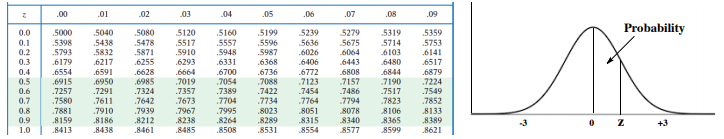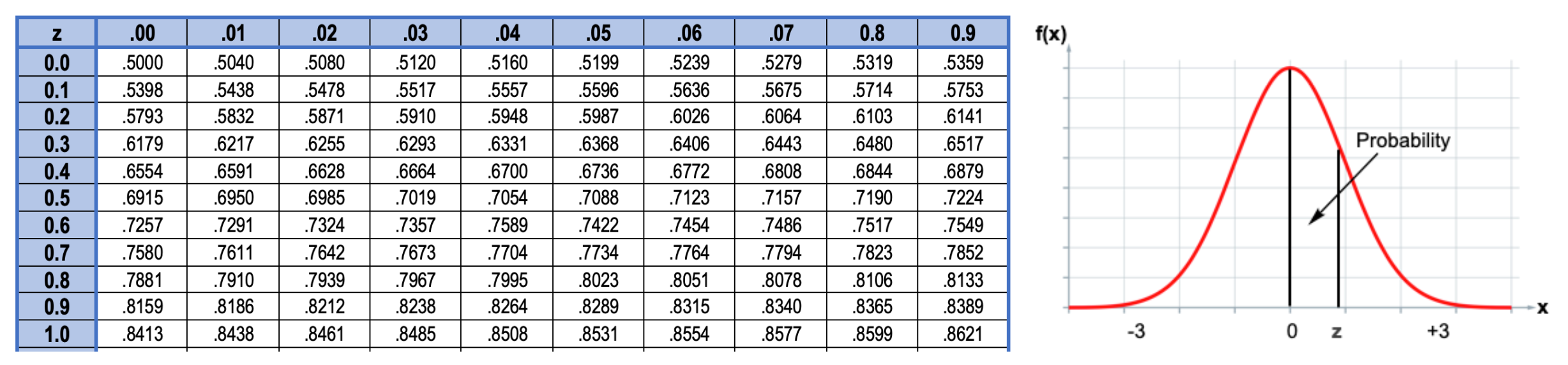Clutch Prep is now a part of Pearson
Ch.4 + 5 - Statistics, Quality Assurance and Calibration MethodsWorksheetSee all chapters

# Hypothesis Testing (t-Test)

See all sections
Sections
Mean Evaluation
The Gaussian Distribution
Confidence Intervals
Hypothesis Testing (t-Test)
Analysis of Variance (f-Test)
Detection of Gross Errors

The t-Test is used to measure the similarities and differences between two populations.

###### The t-test

Concept #1: In order to measure the similarities and differences between populations we utilize a t score

Example #1: A student wishing to calculate the amount of arsenic in cigarettes decides to run two separate methods in her analysis. The results (shown in ppm) are shown below

Sample            Method 1       Method 2

1                      110.5                104.7

2                       93.1                  95.8

3                       63.0                  71.2

4                       72.3                  69.9

5                      121.6                118.7

Is there a significant difference between the two analytical methods under a 95% confidence interval?

Example #2: You want to determine if concentrations of hydrocarbons in seawater measured by fluorescence are significantly different than concentrations measured by a second method, specifically based on the use of gas chromatography/flame ionization detection (GC-FID).  You measure the concentration of a certified standard reference material (100.0 µM) with both methods seven (n=7) times.  Specifically, you first measure each sample by fluorescence, and then measure the same sample by GC-FID.  The concentrations determined by the two methods are shown below.

Sample            Fluorescence             GC-FID

1                       100.2                            101.1

2                       100.9                            100.5

3                        99.9                              100.2

4                       100.1                            100.2

5                       100.1                            99.8

6                       101.1                            100.7

7                       100.0                            99.9

Calculate the appropriate t-statistic to compare the two sets of measurements.

Example #3: A sample of size n = 100 produced the sample mean of 16. Assuming the population deviation is 3, compute a 95% confidence interval for the population mean.

Practice: The average height of the US male is approximately 68 inches. What is the probability of selecting a group of males with average height of 72 inches or greater with a standard deviation of 5 inches?